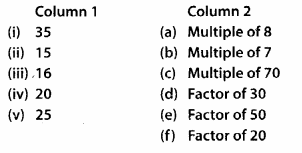# MP Board Class 6th Maths Solutions Chapter 3 Playing With Numbers Ex 3.1

## MP Board Class 6th Maths Solutions Chapter 3 Playing With Numbers Ex 3.1

Question 1.
Write all the factors of the following numbers:
(a) 24
(b) 15
(c) 21
(d) 27
(e) 12
(f) 20
(g) 18
(h) 23
(i) 36
Solution:
(a) 24 =1 × 24 = 2 × 12 = 3 × 8 = 4 × 6 = 6 × 4
∴ Factors of 24 are 1, 2, 3, 4, 6, 8, 12 and 24
(b) 15 = 1 × 15 = 3 × 5 = 5 × 3
∴ Factors of 15 are 1, 3, 5 and 15
(c) 21 = 1 × 21 = 3 × 7 = 7 × 3
∴ Factors of 21 are 1, 3, 7 and 21
(d) 27 = 1 × 27 = 3 × 9 = 9 × 3
∴ Factors of 27 are 1, 3, 9 and 27
(e) 12 = 1 × 12 = 2 × 6 = 3 × 4 = 4 × 3 = 6 × 2
∴ Factors of 12 are 1, 2, 3, 4, 6 and 12
(f) 20 = 1 × 20 = 2 × 10 = 4 × 5 = 5 × 4
∴ Factors of 20 are 1, 2, 4, 5, 10 and 20
(g) 18 = 1 × 18 = 2 × 9 = 3 × 6 = 6 × 3 = 9 × 2
∴ Factors of 18 are 1, 2, 3, 6, 9 and 18
(h) 23 = 1 × 23
∴ Factors of 23 are 1 and 23,
(i) 36 = 1 × 36 = 2 × 18 = 3 × 12 = 4 × 9 = 6 × 6
∴ Factors of 36 are 1, 2, 3, 4, 6, 9, 12, 18 and 36Question 2.
Write first five multiples of:
(a) 5
(b) 8
(c) 9
Solution:
(a) 5 × 1 = 5, 5 × 2 = 10, 5 × 3 = 15, 5 × 4 = 20, 5 × 5 = 25
∴ First five multiples of 5 are 5,10,15, 20, 25.
(b) 8 × 1 = 8, 8 × 2 = 16, 8 × 3 = 24, 8 × 4 = 32,
8 × 5 = 40
∴ First five multiples of 8 are 8,16, 24, 32, 40.
(c) 9 × 1 = 9, 9 × 2 = 18, 9 × 3 = 27, 9 × 4 = 36,
9 × 5 = 45
∴ First five multiples of 9 are 9, 18, 27, 36, 45.Question 3.
Match the items in column 1 with the items in column 2.Solution:
(i) ➝
(b) ;
(ii) ➝ (d);
(iii) ➝ (a);
(iv) ➝ (f);
(v) ➝ (c)
(a) Multiples of 8 are 8, 16, 24, 32, 40, ….
(b) Multiples of 7 are 7, 14, 21, 28, 35, …..
(c) Multiples of 70 are 70,140, 210, ……
(d) Factors of 30 are 1, 2, 3, 5, 6, 10,15, 30.
(e) Factors of 50 are 1, 2, 5, 10, 25.
(f) Factors of 20 are 1, 2, 4, 5, 10, 20.Question 4.
Find all the multiples of 9 upto 100.
Solution:
Multiples of 9 upto 100 are 9, 18, 27, 36, 45, 54, 63, 72, 81, 90, 99